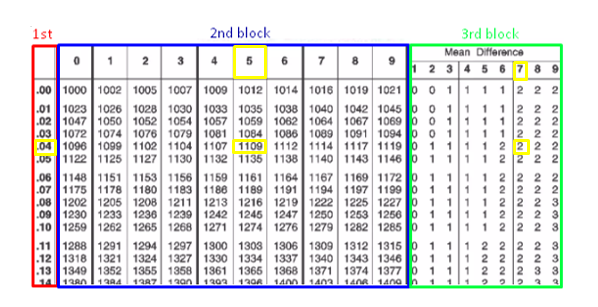# An introduction to Antilogarithm: Defined and Explained with Examples

Before understanding anti-logarithms, you should know about logarithms. Many years ago, when the calculator or the computer was not invented, the calculation of larger numbers was too difficult.

In 1614 first time Mathematician John Napier introduced Logarithm and to find a logarithm he published the logarithm table. In 1620, the anti-logarithm table was represented by Jobst Burgi. After these discoveries of the logarithm, the calculation becomes easier.

The concept of anti-logarithm is the same as the concept of the square and square root being inverse of each other. Such that square of 2 is 4 and the square root of 4 is 2. In this article, we are going to discuss the relationship between anti-logarithms and their properties. At last, we will solve some examples.

## Relation between Logarithms and Antilogarithms

Today logarithms consider the most useful tool to find an unknown variable that occurs in an exponent (power) such that 10p = 9 we will apply logarithmic laws to this problem. Logarithms are defined as; if bx = z then “x” is called the logarithms of “z” to the base “b” mathematically it is written as logbz = x

Where z > 0 and a > 0 (but not equal to 1) the relation bx = z and logbz = x are equal. For example, 42 = 16 is equal to log416 = 2.

The inverse of a Logarithm is known as Antilogarithm.  If log y = x then Antilog x = y. Antilogarithm is meaningless without a logarithm. For example logarithms of 21.12 is 1.3247 and the antilogarithms of 1.3247 are 21.12.

Note: Logarithms of negative value don’t exist.

## Properties of Logarithms

We can’t solve complicated logarithms problems without applying logarithms properties so you should remember these Properties of logarithms. Some properties of logarithms are given below;

1. log a (A ×B) = log b A + log b B
2. log a (A / B) = log b A – log b B
3. log by × log ab = [log y / log b] × [log b / log a]
4. log ay = log by × log ab
5. log a y n = n log a y

## Definition of characteristics and Mantissa

If you want to find antilogarithms by using an antilog table then you should know about characteristics and Mantissa. Logarithms of any number have two parts;

1. Characteristics
2. Mantissa

### Characteristics

In the logarithms of any number, the integral part is called characteristics. If we take the logarithms of any number which is greater than 1 then we get positive characteristics. But if we take logarithms of any number which is less than 1 we get negative characteristics.

For example

Log (21.12) = 1.3247

21.12 is greater than 1 so its characteristic is positive. The integral part of Log 21.12 is 1 which is characteristic.

### Mantissa

In the logarithms of any number, the decimal part is called Mantissa. It contains four digits. It must be positive. In the above example, .3247 is Mantissa.

## Method to find Antilogarithms

We can find antilogarithms by using the calculator to take (10) x where x is the given number

Example 1.

Calculate the antilogarithm of the number 1.2345

Solution

1.2345 is the given number so take 101.2345 = 17.16

The Antilogarithm of the number 1.2345 is 17.16

Example 2.

Find the Antilogarithm of the number 1.0457 by using the Anti-logarithm table.

Solution

Step 1: First identify the characteristic and mantissa. As we know that the integral part is characteristics and the decimal part is the mantissa. Here

Characteristic = 1

Mantissa = .0457

Step 2: you can observe that the antilog table is split into three blocks. Take mantissa and see the First 2 digits (.04) in 1st block, 3rd digit (5) in 2nd block, and 4th digit (7) see in 3rd block.Step 3: Now add the value of the 2nd and 3rd block i.e. 1109 + 2 = 1111

Step 4: If the characteristic is positive then add 1 to it and if the characteristic is negative then subtract it from 1. Now the characteristic is one so after adding 1 we get 2, put the decimal point after two digits from the right.

Antilog 1.0457 = 11.11

The antilogarithm of different values along with the base can be evaluated with the help of an antilog calculator by Allmath (https://www.allmath.com/antilog-calculator.php) to get the step-by-step solution in no time.

Example 3.

Solve 7.4567 × 4.3445 by using a logarithm.

Solution

Let

x = 7.4567 × 4.3445

Taking logs on both sides

Log x = log (7.4567 × 4.3445)

Using the logarithm Product rule

Log x = log 7.4567 + log 4.3445

Calculate the log by using the logarithm table. The logarithm table is divided into three blocks.  You can look at the decimal point and not need to move so characteristics are zero for both.

So the first 2 digits of decimal places find in the 1st block and 3rd digit of the decimal place find in the 2nd block and 4th digit of the decimal place find in the 3rd block. Then add the value of the 2nd and 3rd blocks.  After taking the logarithm we get

Log x = 0.8725 + 0.6379

Log x = 1.5104

Taking Antilog on both sides

Antilog (log x) = Antilog (1.5104)

x = Antilog (1.5104)

By using an antilog table we get

x = 32.39

## Conclusion

In this article, we have discussed the history of logarithms and Antilogarithms. John Napier and Jobst Burgi were great mathematicians who gave the concept of logarithms and anti-logarithms. The definitions of logarithms and anti-logarithms are defined in this article. All terms related to anti-logarithms are also covered with examples, Such that characteristics and mantissa.

The important laws of logarithms to calculate difficult problems are discussed in this article. We learned in the example section, how to find antilog by using the antilog table with a step-by-step solution. After reading this article you will be able to calculate the Anti-logarithm problems.